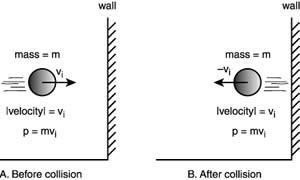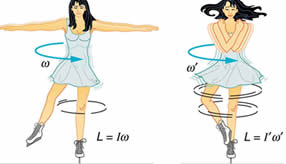# Difference between Momentum and Angular Momentum

##### Key Difference:  Linear Momentum is basically used to measure the quantity of motion of an object. It can be simply referred to as mass in motion. Linear moment is a product of mass and velocity, and can be generated in both vertical and horizontal directions. Angular momentum can be considered as the rotational analog of linear momentum. Angular momentum considers both the moment of inertia about an axis as well as the angular velocity about that axis.Linear momentum or just momentum is basically used to measure the quantity of motion of an object. It can be simply referred to as mass in motion. It is possessed by all the objects which are moving, as all the objects contain mass. It depends upon two variables – the mass of the object and the velocity with which the object is moving.

Simply, it can be defined as the product of mass and velocity. The standard unit of measurement for momentum is kg m/s. It is a vector quantity and the direction is same that of the direction of the object.Angular momentum can be considered as the rotational analog of linear momentum. It can also be referred to as the momentum of rotation. Any object which moves around a point has angular momentum. Angular momentum of a rigid body possessing moment of Inertia I rotating with angular velocity w   can be obtained by using following formula =

L = Iw, I = Moment of Inertia and w= Angular velocity

The unit of angular moment is kgm^2/s

Both linear and angular momentums are vector entities. Angular momentum can also be described in terms of linear momentum as it is the vector product between the position vector and the linear momentum. Angular momentum is dependent on the magnitude as well as the direction of the position and linear momentum vectors.

Comparison between Momentum and Angular Momentum:

 Momentum Angular Momentum Definition Linear momentum or just momentum is basically used to measure the quantity of motion of an object. It can be simply referred to as mass in motion. Angular momentum can be considered as the rotational analog of linear momentum. It can also be referred to as the momentum of rotation. Unit of measurement The unit for momentum is kg m/s. The unit for momentum is kg m^2/s. Formula Momentum = mass * velocity Angular momentum = Moment of inertia for mass * angular velocity Properties It is a vector quantity It has same direction as velocity It helps in understanding collisions It is also a vector quantity It points in the same direction as the moment of inertia for mass Conservation rule It is conserved when there are no external forces acting. It is conserved if no net torques are involved.

Image Courtesy: yaldex.com, physics.stackexchange.com